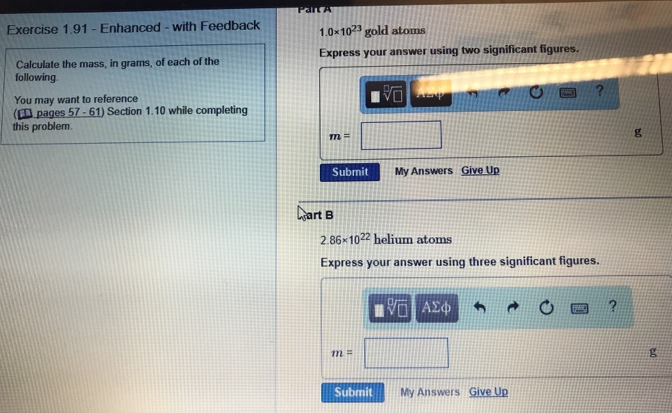# Problem: Calculate the mass, in grams, of each of the following. Part A1.0 x 1023 gold atomsExpress your answer using three significant figures.Part B 2.86 x 1022 helium atoms Express your answer using three significant figures.

🤓 Based on our data, we think this question is relevant for Professor Shay's class at BGSU.

###### FREE Expert Solution###### Problem Details

Calculate the mass, in grams, of each of the following.

Part A

1.0 x 1023 gold atoms